Loading, please wait...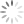# Most Used SQL Queries | Important SQL Queries

In this article, we will look upon the various important and widely used SQL Queries used in SQL Server to interact with the database in different ways

# Important SQL Queries

### 1. Create table:

``````create table table_name
(

id int,
name varchar(20),
salary int

)``````

### 2. Create a table with a primary key

``````create table table_name
(

id int primary key,
name varchar(20),
salary int

)  ``````

Table Designer:### 3. Insert multiple rows in a single column

``````insert into table_name (id,name,salary) values (1,'ritika', 45000), (2,'rishi', 43000), (3,'rahul',54000);

select * from table_name``````

Table Designer:### 4. Update all records

``````update table_name set salary=40000

select * from table_name``````

Table Designer: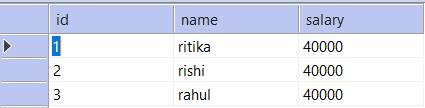### 5. Create a view

``````create view

view_name as select id,name,salary from table_name where (salary < 50000)

select * from view_name``````

Table View:### 6. Get all column names from a table :

``````select column_name from INFORMATION_SCHEMA.COLUMNS

where TABLE_NAME= 'employee1' ;``````

Table View:### 7. Get all user-created tables:

``select name from sys.objects where type='U' ;``

Result View: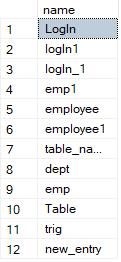### 8. Get all views names:

``select * from sys.views``

### 9. Get all stored procedures name from the database:

``select * from sys.procedures``

### 10. Counting rows of all tables at once:

``````select [table_name] = so.name, [rowcount] = MAX (si.rows)
from sysobjects so, sysindexes si where so.xtype = 'U' and si.id = OBJECT_ID(so.name)group by so.name order by 2 desc``````

Result View: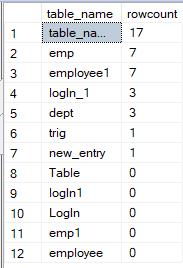### 11.  Retrieve a list of all database names

``exec sp_databases``

Result View: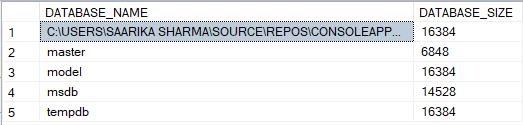### 12. Check default language of SQL server

``select @@LANGUAGE as DefaultLanguage``

Result View: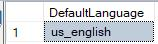### 13. Check server name

``select @@SERVERNAME as ServerName``

### 14. Add columns to the existing table:

``````alter table {table_name} add {columnName} {data_type}

example:

alter table table_name add location varchar(20)

``````

Result View:### 15. Remove column from a table:

``````alter table {table_name}

drop column {column_name}``````

### 16. Rebuild all index of the database

``exec sp_MSforeachtable @command1 = "print '?' DBCC DBREINDEX ('?' , ' ' , 80)"``

Result View: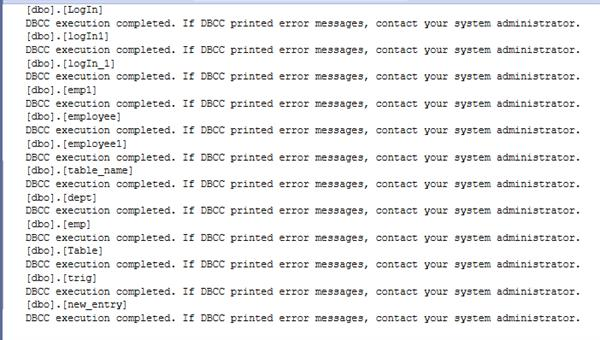### 17. Find bytesize of all tables in database

``select sob.name as table_name, sum(sys.length ) as [size_table(bytes)] from sysobjects sob, syscolumns sys where sob.xtype = 'U' and sys.id = sob.id group by sob.name``

Result View: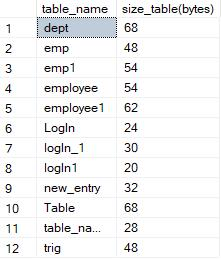### 18. List of the primary key and foreign key for the whole database

``select distinct constraint_name as [constraint], table_schema as [schema], table_name as [tablename] from INFORMATION_SCHEMA.KEY_COLUMN_USAGE``

Result View: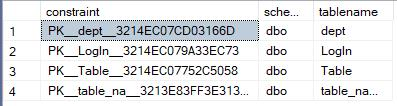### 19. Delete duplicate rows:

``````delete from table_name where id not in
( select max(id) from table_name group by duplicateColumn1 , duplicateColumn2)``````

### 20. Reseed identity of all tables

``````exec sp_MSforeachtable '

if objectproperty(object_id("?") , "tableHasIdentity") = 1

DBCC checkIDENT ("?" , RESEED , 0) '``````

### 21. Disable all constraints of a table:

``alter table table_name nocheck constraint all``

### 22. Get current language ID

``select @@LANGID as 'language id'``

Result View:### 23. Get precision level used by decimal and numeric as current set in the server

``select @@MAX_PRECISION as 'MAX_Preicision'``

Result View: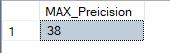### 24. Get the name of register key under which SQL server is running

``select @@SERVICENAME as 'Service_Name'``

Result View: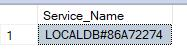### 25. Get session Id of the current user process

``select @@SPID as 'Session_Id'``

Result View: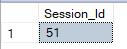### 26. Get the current value of text_Size

``````set TEXTSIZE 0;

select @@TEXTSIZE as 'Text_Size'

set TEXTSIZE 1324;

select @@TEXTSIZE as 'Text_Size'

set TEXTSIZE 11456;

select @@TEXTSIZE as 'Text_Size'``````

Result View: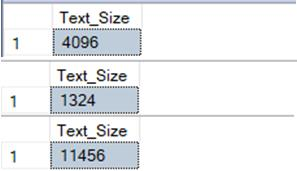### 27. Retrieve free space of the hard disk

``````exec master..xp_fixeddrives
``````

Result View: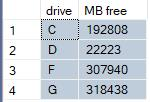### 28. Disable a particular trigger

``alter table table_name disable trigger trigger_name``

### 29. Enable all Triggers:

``alter table table_name enable trigger trigger_name``

### 30. Disable all triggers for the database:

``````use database_name

exec sp_MSforeachtable "alter table ? disable trigger a11"``````

### 31. Enable all triggers for the database:

``````use database_name

exec sp_MSforeachtable "alter table ? enable trigger a11"``````

### 32. Get all columns of a specific datatype:

``````select object_name(c.object_ID) as table_name , c.name as column_name

from sys.columns as c

join sys.types as t on c.user_type_id = t.user_type_id

where t.name = 'DataType'  ``````

### 33. Get all nullable columns of a table

``````select object_name (c.object_id) as table_name , c.name as column_name

from sys.columns as c

join sys.types as t on c.user_type_id = t.user_type_id

where c.is_nullable = 0 and object_name(c.object_id) = 'table_name'``````

Result View: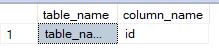### 34. Get all tables that don’t have a primary key

``select name as table_name from sys.tables where OBJECTPROPERTY(object_id,'tableHasPrimaryKey') = 0 order by table_name;``

Result View:### 35. Get all tables that do not have a foreign key

``select name as table_name from sys.tables where OBJECTPROPERTY(object_id,'tableHasForeignKey') = 0 order by table_name;``

Result View: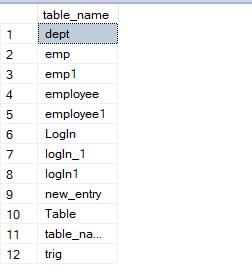### 36. Get all tables that don’t have an identity column

``select name as table_name from sys.tables where OBJECTPROPERTY(object_id,'tableHasIdentity') = 0 order by table_name;``

Result View: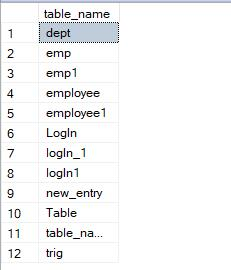### 37. Get the first date of the current month

``select convert(varchar(20) , dateAdd(day, - (day(getDate())) +1 , getDate()), 105) first_data_current_month;``

Result View: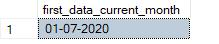### 38. Get the last date of the previous month

``select convert(varchar(20) , dateAdd(day, - (day(getDate())) , getDate()), 105) last_date_previous_month;``

Result View: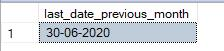### 39. Get the first date of next month

``select convert(varchar(20) , dateAdd(day, - (day(getDate())) , dateAdd (month , 1, GetDate()) +1 ) , 105 ) first_date_Next_month;``

Result View: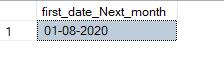### 40. Swap the values of two columns:

``update table_name set column1=column2 , column2 = column1``